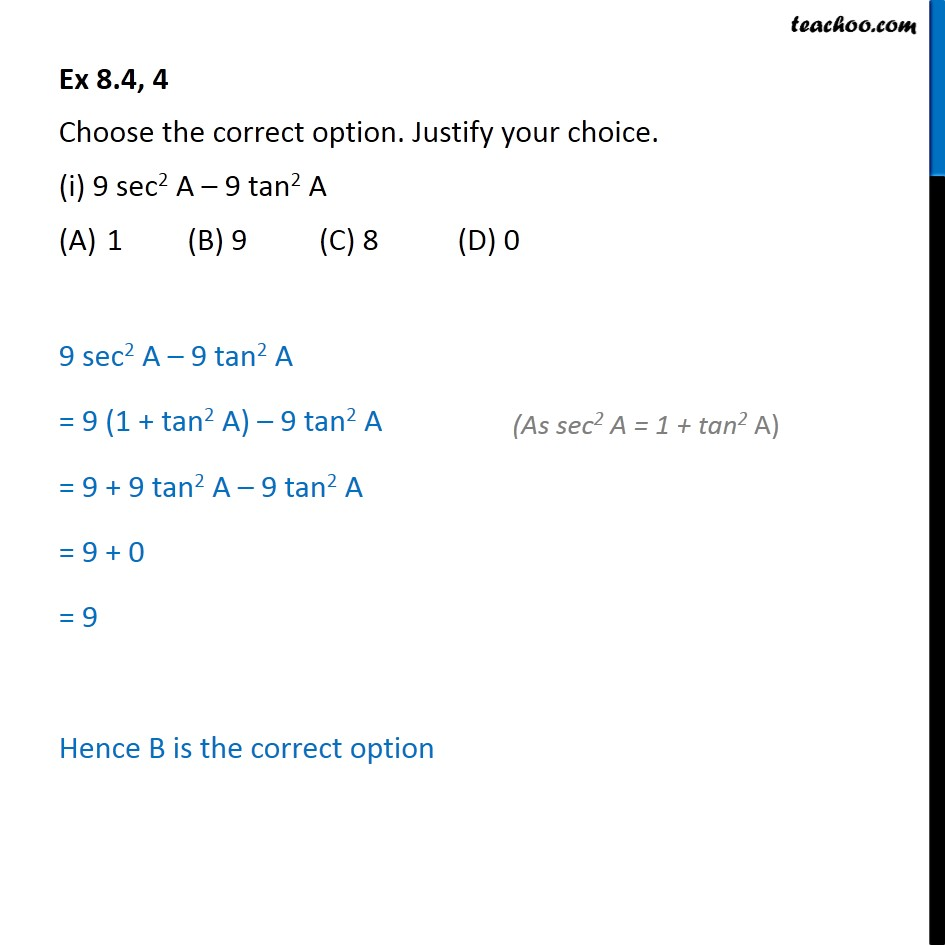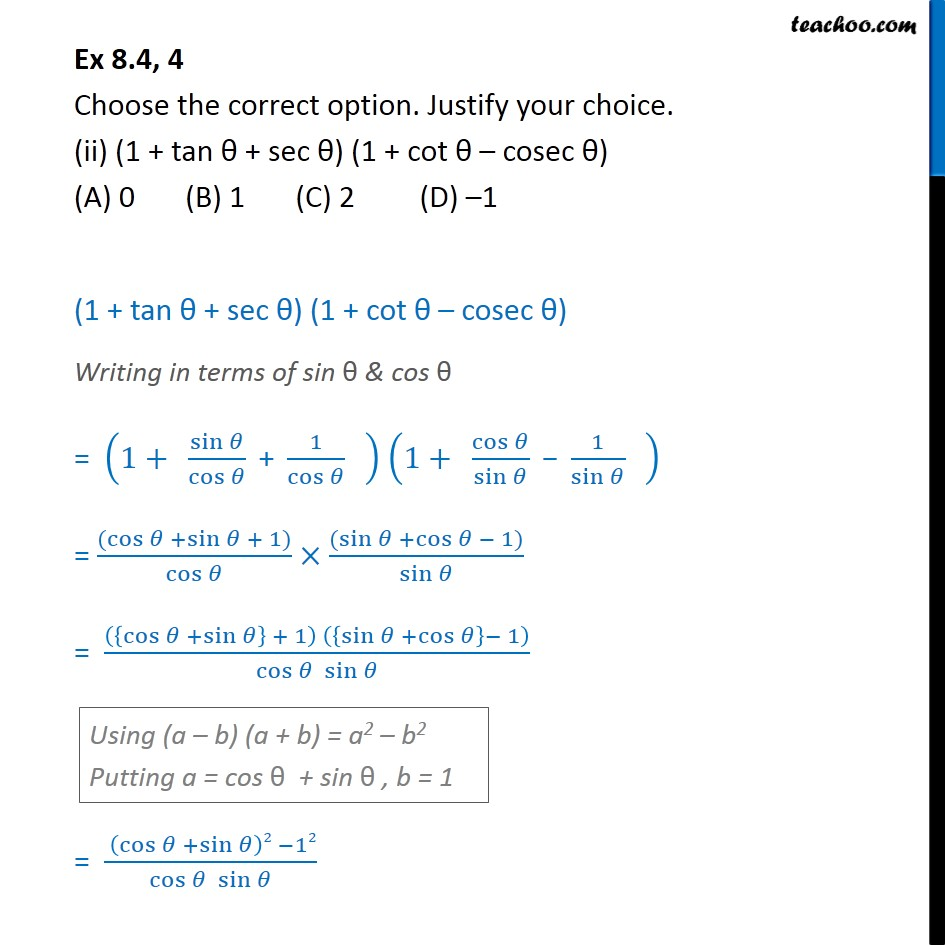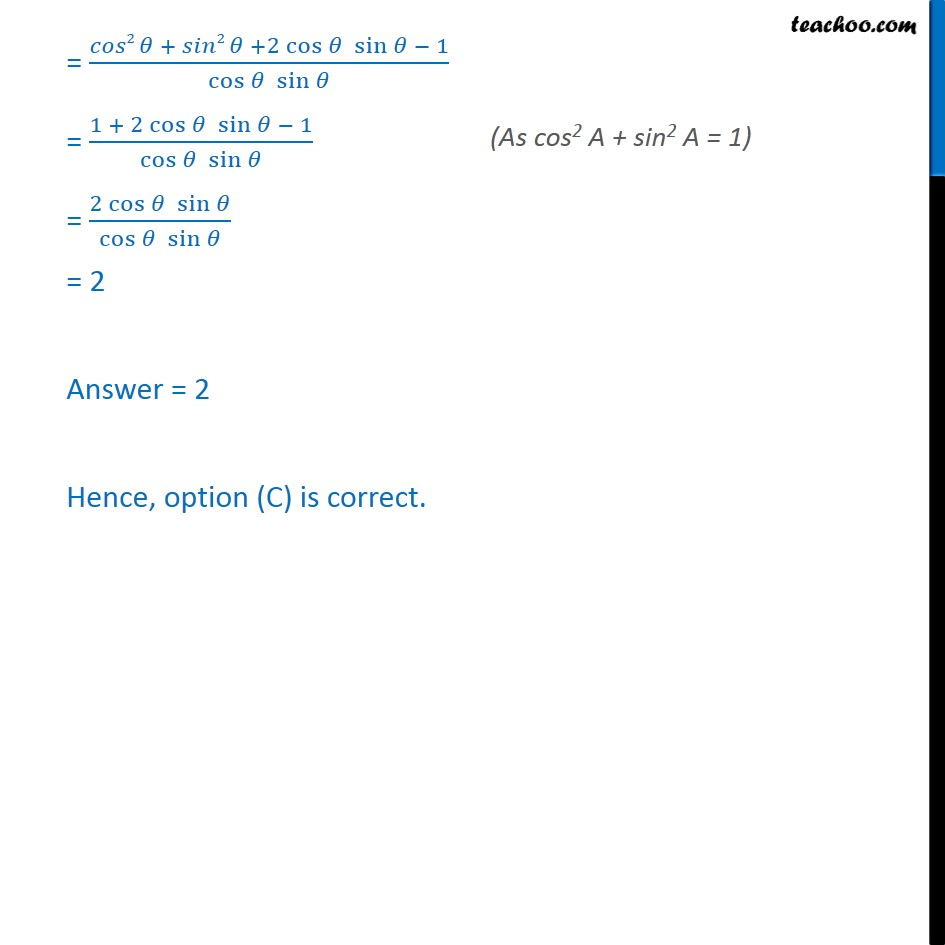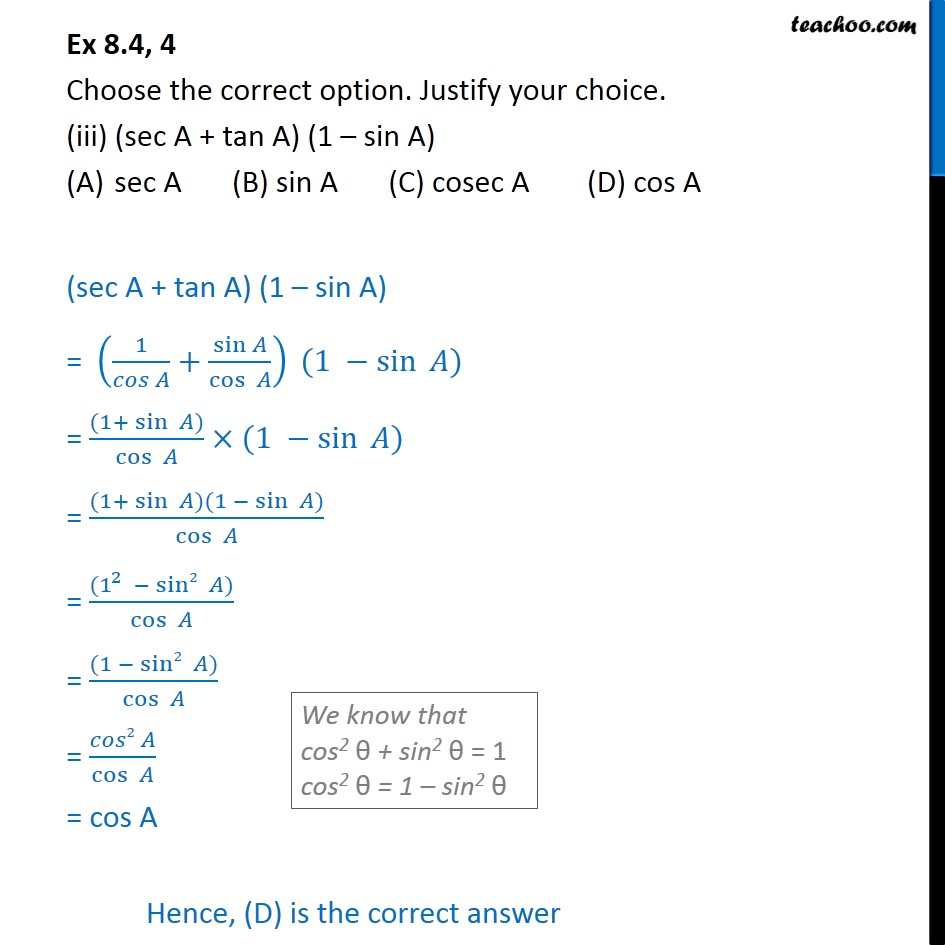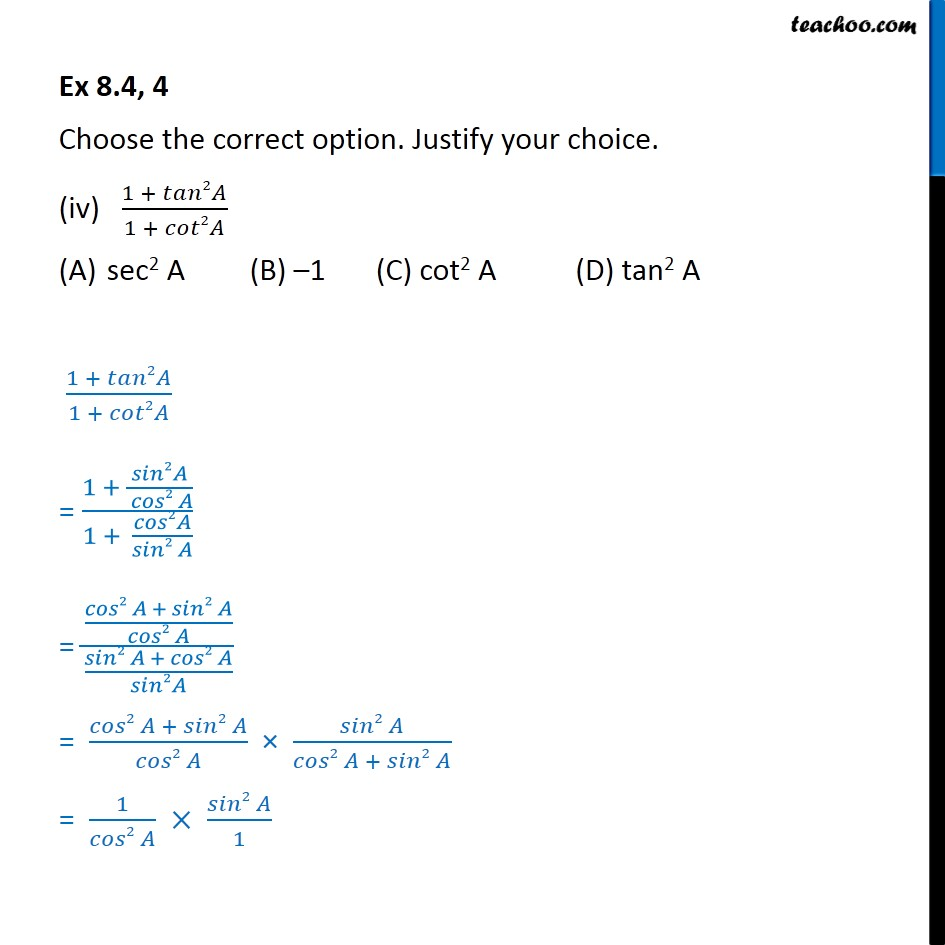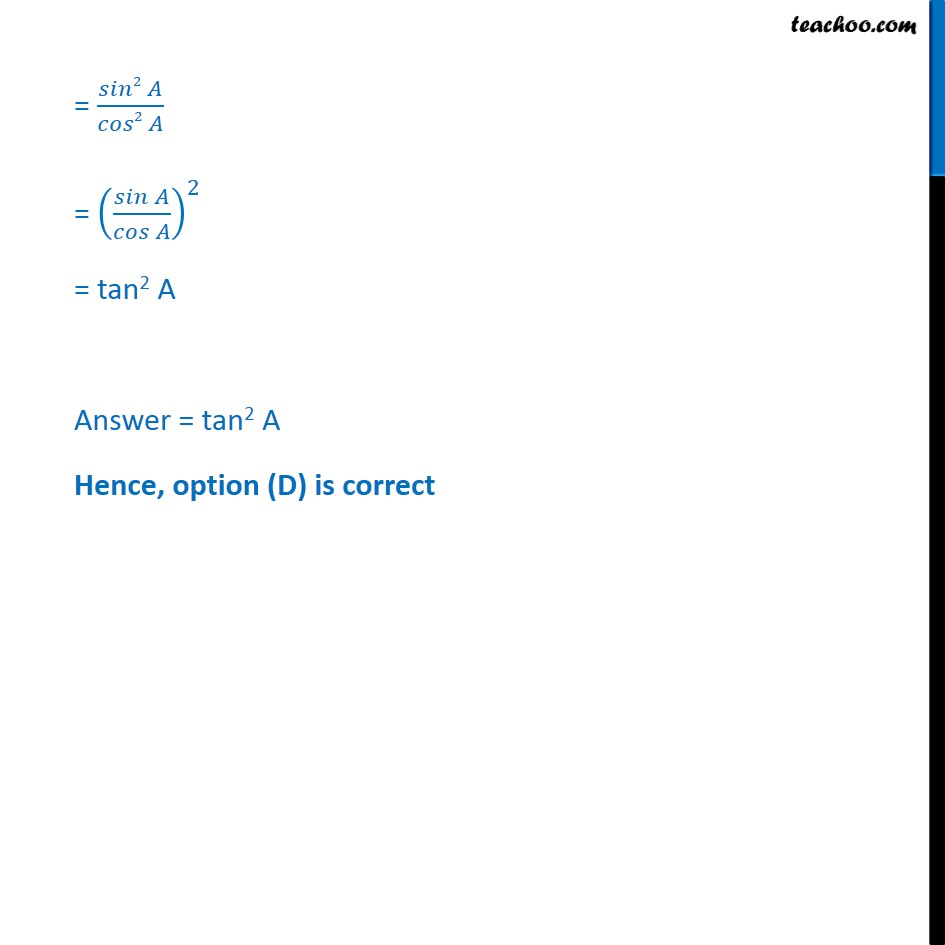Subscribe to our Youtube Channel - https://you.tube/teachoo

1. Chapter 8 Class 10 Introduction to Trignometry
2. Serial order wise
3. Ex 8.4

Transcript

Ex 8.4, 4 Choose the correct option. Justify your choice. (i) 9 sec2 A – 9 tan2 A 1 (B) 9 (C) 8 (D) 0 9 sec2 A – 9 tan2 A = 9 (1 + tan2 A) – 9 tan2 A = 9 + 9 tan2 A – 9 tan2 A = 9 + 0 = 9 Hence B is the correct option Ex 8.4, 4 Choose the correct option. Justify your choice. (ii) (1 + tan θ + sec θ) (1 + cot θ – cosec θ) (A) 0 (B) 1 (C) 2 (D) –1 (1 + tan θ + sec θ) (1 + cot θ – cosec θ) Writing in terms of sin θ & cos θ = (1+" " sin⁡𝜃/cos⁡𝜃 " + " 1/cos⁡𝜃 " " ) (1+" " cos⁡𝜃/sin⁡𝜃 " − " 1/sin⁡𝜃 " " ) = ((cos⁡𝜃 +sin⁡𝜃 + 1))/cos⁡𝜃 × ((sin⁡𝜃 +cos⁡𝜃 − 1))/sin⁡𝜃 = (({cos⁡𝜃 +sin⁡𝜃 } + 1) ({sin⁡𝜃 +cos⁡𝜃 }− 1))/(cos⁡𝜃 sin⁡𝜃 ) = ( (cos⁡𝜃 +sin⁡𝜃 )2 −12)/(cos⁡𝜃 sin⁡𝜃 ) = (𝑐𝑜𝑠2 𝜃 + 𝑠𝑖𝑛2 𝜃 +2 cos⁡𝜃 sin⁡𝜃 − 1)/(cos⁡𝜃 sin⁡𝜃 ) = (1 + 2 cos⁡𝜃 sin⁡𝜃 − 1)/(cos⁡𝜃 sin⁡𝜃 ) = (2 cos⁡𝜃 sin⁡𝜃)/(cos⁡𝜃 sin⁡𝜃 ) = 2 Answer = 2 Hence, option (B) is correct. Ex 8.4, 4 Choose the correct option. Justify your choice. (iii) (sec A + tan A) (1 – sin A) sec A (B) sin A (C) cosec A (D) cos A (sec A + tan A) (1 – sin A) = (1/(𝑐𝑜𝑠 𝐴)+sin⁡𝐴/cos⁡〖 𝐴〗 ) (1 −sin⁡〖 𝐴)〗 = ((1+ sin⁡〖 𝐴)〗)/cos⁡〖 𝐴〗 ×(1 −sin⁡〖 𝐴)〗 = ((1+ sin⁡〖 𝐴)(1 −〖 sin〗⁡〖 𝐴)〗 〗)/cos⁡〖 𝐴〗 = ((1^2 −〖 sin2〗⁡〖 𝐴)〗)/cos⁡〖 𝐴〗 = ((1 −〖 sin2〗⁡〖 𝐴)〗)/cos⁡〖 𝐴〗 = (𝑐𝑜𝑠2 𝐴)/cos⁡〖 𝐴〗 = cos A Hence, (D) is the correct answer Ex 8.4, 4 Choose the correct option. Justify your choice. (iv) (1 + 𝑡𝑎𝑛2𝐴)/(1 + 𝑐𝑜𝑡2𝐴) sec2 A (B) –1 (C) cot2 A (D) tan2 A (1 + 𝑡𝑎𝑛2𝐴)/(1 + 𝑐𝑜𝑡2𝐴) = (1 + 𝑠𝑖𝑛2𝐴/( 𝑐𝑜𝑠2 𝐴))/(1 + 𝑐𝑜𝑠2𝐴/(𝑠𝑖𝑛2 𝐴)) = ((𝑐𝑜𝑠2 𝐴 + 𝑠𝑖𝑛2 𝐴)/(𝑐𝑜𝑠2 𝐴))/((𝑠𝑖𝑛2 𝐴 + 𝑐𝑜𝑠2 𝐴)/𝑠𝑖𝑛2𝐴) = (𝑐𝑜𝑠2 𝐴 + 𝑠𝑖𝑛2 𝐴)/(𝑐𝑜𝑠2 𝐴) × (𝑠𝑖𝑛2 𝐴)/(𝑐𝑜𝑠2 𝐴 + 𝑠𝑖𝑛2 𝐴) = 1/(𝑐𝑜𝑠2 𝐴) × (𝑠𝑖𝑛2 𝐴)/1 = (𝑠𝑖𝑛2 𝐴)/(𝑐𝑜𝑠2 𝐴) = ((𝑠𝑖𝑛 𝐴)/(𝑐𝑜𝑠 𝐴))^2 = tan2 A Answer = tan2 A Hence, option (D) is correct

Ex 8.4